# Element structure of dihedral group:D8

View element structure of particular groups | View other specific information about dihedral group:D8

We denote the identity element by$e$. The dihedral group$D_8$, sometimes called$D_4$, also called the dihedral group of order eight or the dihedral group acting on four elements, is defined by the following presentation:$\langle x,a| a^4 = x^2 = e, xax^{-1} = a^{-1}\rangle$

Below is the multiplication table, with the row element multiplied on the left and the column element on the right.

## Summary

Item Value
order of the whole group (total number of elements) 8
conjugacy class sizes 1,1,2,2,2
maximum: 2, number of conjugacy classes: 5, lcm: 2
order statistics 1 of order 1, 5 of order 2, 2 of order 4
maximum: 4, lcm (exponent of the whole group): 4

## Elements

Below, we list all the elements, also giving the interpretation of each element under the geometric description of the dihedral group as the symmetries of a 4-gon, and for the corresponding permutation representation (see D8 in S4). Note that for different conventions, one can obtain somewhat different correspondences, so this may not match up with other correspondences elsewhere:

Element in terms of$a$ and$x$ Geometric description Permutation on vertices Order of the element$e$ (identity element) does nothing, i.e., leaves the square invariant$()$ 1$a$ rotation by angle of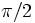$\pi/2$ (i.e.,$90\,^\circ$) counterclockwise$(1,2,3,4)$ 4$a^2$ rotation by angle of$\pi$ (i.e.,$180\,^\circ$), also called a half turn$(1,3)(2,4)$ 2$a^3$ rotation by angle of$3\pi/2$ (i.e.,$270\,^\circ$) counter-clockwise, or equivalently, by$\pi/2$ (i.e.,$90\,^\circ$) clockwise$(1,4,3,2)$$x$ reflection about the diagonal joining vertices "2" and "4"$(1,3)$ 2$ax = xa^3$ reflection about the line joining midpoints of opposite sides "14" and "23"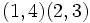$(1,4)(2,3)$ 2$a^2x$ reflection about the diagonal joining vertices "1" and "3"$(2,4)$ 2$a^3x = xa$ reflection about the line joining midpoints of opposite sides "12" and "34"$(1,2)(3,4)$ 2

## Conjugacy class structure

FACTS TO CHECK AGAINST FOR CONJUGACY CLASS SIZES AND STRUCTURE:
Divisibility facts: size of conjugacy class divides order of group | size of conjugacy class divides index of center | size of conjugacy class equals index of centralizer
Bounding facts: size of conjugacy class is bounded by order of derived subgroup
Counting facts: number of conjugacy classes equals number of irreducible representations | class equation of a group
Conjugacy class Size of conjugacy class Order of elements in conjugacy class Centralizer of first element of class$\! \{ e \}$ 1 1 whole group$\! \{ a^2 \}$ 1 2 whole group$\! \{ x,a^2x \}$ 2 2$\{ e, a^2, x, a^2x \}$ -- one of the Klein four-subgroups of dihedral group:D8$\! \{ ax, a^3x \}$ 2 2$\{ e, a^2, ax, a^3x \}$ -- one of the Klein four-subgroups of dihedral group:D8$\! \{ a, a^3 \}$ 2 4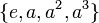$\{ e, a, a^2, a^3 \}$ -- the cyclic maximal subgroup of dihedral group:D8

The equivalence classes up to automorphisms are:

Equivalence class under automorphisms Size of equivalence class Number of conjugacy classes in it Size of each conjugacy class$\! \{ e \}$ 1 1 1$\! \{ a^2 \}$ 1 1 1$\! \{ x, ax, a^2x, a^3x \}$ 4 2 2$\! \{ a, a^3 \}$ 2 1 2

### Convolution algebra on conjugacy classes$\{ e \}$$\{ a^2 \}$$\{ x,a^2x \}$$\{ ax, a^3x \}$$\{ a, a^3 \}$$\{ e \}$$\{ e \}$$\{ a^2 \}$$\{ x,a^2x \}$$\{ ax, a^3x \}$$\{ a, a^3 \}$$\{ a^2 \}$$\{ a^2 \}$$\{ e \}$$\{ x,a^2x \}$$\{ ax, a^3x \}$$\{ a, a^3 \}$$\{ x,a^2x \}$$\{ x,a^2x \}$$\{ x,a^2x \}$$2 \{ e \} + 2 \{ a^2 \}$$2 \{ a,a^3 \}$$2 \{ ax, a^3x \}$$\{ ax, a^3x \}$$\{ ax, a^3x \}$$\{ ax, a^3x \}$$2 \{ a,a^3 \}$$2\{ e \} + 2 \{ a^2 \}$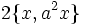$2 \{ x, a^2 x \}$$\{ a,a^3 \}$$\{ a,a^3 \}$$\{ a,a^3 \}$$2\{ ax, a^3x \}$$2 \{ x,a^2x \}$$2 \{ e \} + 2 \{ a^2 \}$

## Order and power information

### Directed power graph

Below is a trimmed version of the directed power graph of the group. There is a dark edge from one vertex to another if the latter is the square of the former. A dashed edge means that the latter is an odd power of the former. We remove all the loops.

### Order statistics

Number Elements of order exactly that number Number of such elements Number of conjugacy classes of such elements Number of elements whose order divides that number Number of conjugacy classes whose element order divides that number
1$\! \{ e \}$ 1 1 1 1
2$\! \{ a^2, x, ax, a^2x, a^3x \}$ 5 3 6 4
4$\! \{ a, a^3 \}$ 2 1 8 5

### Power statistics

Number$d$$d^{th}$ powers that are not$k^{th}$ powers for any larger divisor$k$ of the group order Number of such elements Number of conjugacy classes of such elements Number of$d^{th}$ powers Number of conjugacy classes of$d^{th}$ powers
1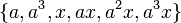$\! \{ a, a^3, x, ax, a^2x, a^3x \}$ 6 3 8 5
2$\! \{ a^2 \}$ 1 1 2 2
4 -- 0 0 1 1
8$\! \{ e \}$ 1 1 1 1